# Use Direct Proportion in Table Form

In this worksheet, students will use direct proportion to find values.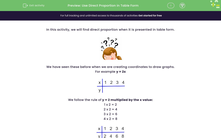Key stage:  KS 3

Curriculum topic:   Ratio, Proportion and Rates of Change

Curriculum subtopic:   Solve Problems Involving Direct and Inverse Proportion

Difficulty level:#### Worksheet Overview

In this activity, we will find direct proportion when it is presented in table form.We have seen these before when we are creating coordinates to draw graphs.

For example y = 2x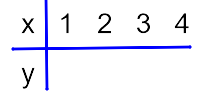We follow the rule of y = 2 multiplied by the x value:

1 x 2 = 2

2 x 2 = 4

3 x 2 = 6

4 x 2 = 8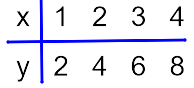The graph can now be plotted!

Does that make sense?Great! In this activity, we will be using the same idea, but we have to find the rule.

Confused? Let's have a look at an example to help!

Example

y is directly proportional to x

Complete the table below: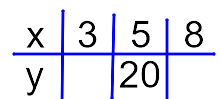We need to look at the table to see where we have the x and the y values.

We can use these to find the rule.

We have x = 5 when y = 20

To find the multiplier, we can do 20 ÷ 5 = 4

So, we can see that y = 4x

or

the y values are 4 times bigger than the x values.

We can now fill in the gaps:

When x = 3, then y = 4 x 3 = 12

When x = 8, then y = 4 x 8 = 32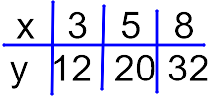Let's try some more of these!

### What is EdPlace?

We're your National Curriculum aligned online education content provider helping each child succeed in English, maths and science from year 1 to GCSE. With an EdPlace account you’ll be able to track and measure progress, helping each child achieve their best. We build confidence and attainment by personalising each child’s learning at a level that suits them.

Get started